# Multiplication Tricks - Find solution within 20 seconds

Today I am going to share quick multiplication tricks that will help you find the solution within 20 seconds. Must read - 10 Coolest Maths tricks

### Multiply by 9,99,999,etc...

56*99=5544
Step 1:Place a zero at the end for each 9 :5600
Step 2 : Subtract the original number from Step 1 like this 5600-56=5544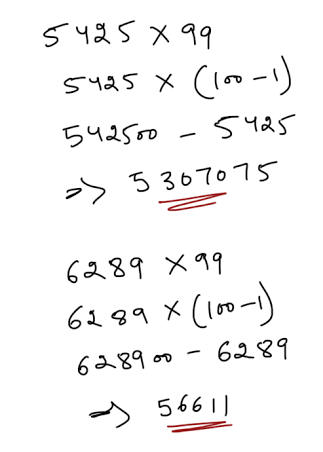### Multiply by 125

68*125=8500
Step 1 :Place three zeros at the end of the number :68000
Step 2: Divide the number from Step 1  by 8:68000/8=8500

64*125 is the same as :
32*250 is the same as
16*500 is the same as
8*1000

64*125
Step 1. Each time you just need to pick 125 multiply it by 8 will get 1000
Step 2. Pick 64 and divide it by 8 will get 8
Step 3. Multiply the results with each other 8* 1000
Hence Solution is 8000
[Hint: Just remember 125*8=1000]

### Multiply two digits numbers ending in 1

51*31=1581
Step 1: Multiply the left most digits : 5*3=15
Step 2: Add the left most digits:5+3=8
Step 3: Places the result from Step 2 next to the result from Step 1:158
Step 4 : Places 1 next to the result from  Step 3 : 1581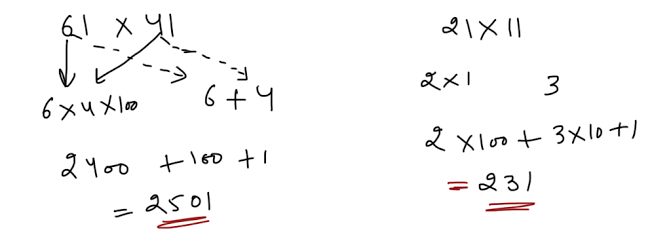### Multiply numbers between 11 and 19

14*18=252
Step 1: Add the larger  number to the right most digit of the other number:18+4=22
Step 2: Put a 0 at the end of the result from step 1:220
Step 3 :Multiply the right most digits of both original numbers : 8*4=32
Step 4:Add Step 2 and step 3 :220+32=252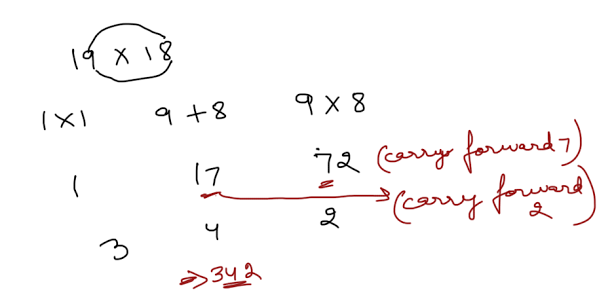### Multiply two digit number by 11

53*11=583
Step 1: Add the both digts of the two digit number:5+3=8
Step 2: Place the result in between both digits : 583

59*11=649
Step 1: 5+9=14
Step 2 : Carry the 1 when the result is greater than 9:5+1=6
Step 3: 649### Multiply by 5

1234 *5 =6170
Step 1 : Divide  the number by 2 :1234/2=617
Step 2: Multiply  the result from Step 1 by 10 : 617*10=6170

### Multiply by 25

18*25=450
Step 1: Divide  the number by 4:18/4
Step 2: Multiply  the number from Step 1 by 100: 4.5 * 100 = 450

### Multiply by 9

56*9=504
Step 1: Multiply the number by 10: 56*10=560
Step 2: Subtract the original number from Step 1: 560-56=504

### Factorization

By Factoring number,you can break down problems into simpler multiplication tasks.Also,you may be able to apply some techniques you learned.

21*33
step 1 : 21*11*3
Step 2: 231*3
Step 3 :693

67*81
Step 1: 67*9*9
Step 2:603*9=5427

28*125=3500
Step 1: 28*125
Step 2: 28*25*5
Step 3:28*(100/4)*5
Step 4:28/4*100*5
Step 5:7*500=3500

### Some Special type

#### When sum of unit digit is 10 and remaining digit is same.

43×47 = 4 × ( 4+1 ) / 3×7
= 4×5/21
20/21
Ans = 2021

72×78 = 7×8 / 2×8
= 56/16
Ans = 5616

104 × 106 = 10× ( 10+1 ) / 4×6
= 10 × 11/24
= 110/24
Ans = 11024

#### When sum of ten's digit is 10 and unit digit is same

46 × 66
= ( 4×6 ) +6 / 6×6
= 24 + 6/36
= 30/ 36
Ans = 3036

83 × 23
= ( 8×2 ) +3 / 3×3
= 19/09
Ans = 1909

92 × 12
= ( 9×1 ) + 2/2 × 2
= 11/04
Ans = 1104

#### When unit digit is 5 in both the numbers and difference between both number is 10.

75 × 65
=  6 × ( 7+1 ) / 75
=  48/75
Ans = 4875

45 × 35
= 3 × ( 4+1 )/75
= 15 /75
Ans = 1575

105 × 95
= 9 × ( 10+1 ) / 75
= 99/75
Ans = 9975

#### Find the unit digit  of 147128 * 138148 ?### Multiply by 11,111,1111....so on

Ques 1. 111111111 ✘ 111111111 = ?
Sol:
No of digits in multiplier = 9
Write in ascending order from left side like this:
987654321
and now 9-1=8
write it in descending order just after it
12345678
now you will get like this:
12345678987654321
hence
111111111111111111 = 12345678987654321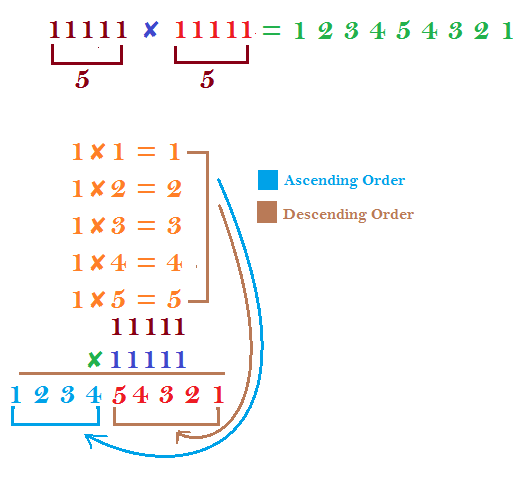Ques 2. 1111111111 ✘ 1111111111 = ?
Sol:
No of digits in multiplier =10
Write in ascending order from left side like this:
10 9 8 7 6 5 4 3 2 1
and now 10-1=9
write it in descending order just after it
1 2 3 4 5 6 7 8 9
and after it just add the carry
1 2 3 4 5 6 7 8/ 9/ 10 9 8 7 6 5 4 3 2 1
8+1/   9+1 / 0
1 2 3 4 5 6 7 9 0 0 9 8 7 6 5 4 3 2 1
now you will get like this:
1234567900987654321
hence
1111111111✘1111111111 = 1234567900987654321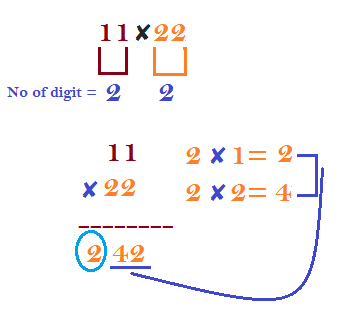Ques 3. 11111112222222 = ?
Sol:
No of digit in the multiplier is 7 then let n=7;
Now Just multiply the digit 2 from 1 to 7 time & arrange them from extreme left to right in ascending order,you will get like this:
14 12 10 8 6 4 2
and now just subtract one from n.like this n=7,so n-1=6.
Multiply the digit 2 from 1 to 6 time & arrange them from just right after it,you will get like this:
2 4 6 8 10 12
Now placing both outcome like this & add the carry
2 4 6 8 10 12 14 12 10 8 6 4 2
8+1/0+1/2+1/4+1/2+1
2 4 6 9 1 3 5 3 0 8 6 4 2

Ques 4. 1111111✘5555555 = ?
Sol:
No of digit = 7
Now Just multiply the digit 5 from 1 to 7 time & arrange them from extreme left to right in ascending order,you will get like this:
35 30 25 20 15 10 5
Just right after it perform same action but in descending order & till 6 times only.like this:
5 10 15 20 25 30
Now placing together ,just add the carry
5 10 15 20 25 30 35 30 25 20 15 10 5
6 1 7 2 8 3 8 2 7 1 6 0 5
11111115555555=6172838271605#### What's trending in BankExamsToday

Smart Prep Kit for Banking Exams by Ramandeep Singh - Download here1.thnku sir....

1.2.thank u sir

3.thanks sir:)

4.thank u sir..
Sir any idea about Lakshmi vilas bank PO exam??

5.Watz der n a nameJune 2, 2015 at 5:44 PM

Thanku soo much sir...really this wll save time!!!thanks alot

6.THANKS A TON

7.sir, we are paying a lot of thanks to u.

8.wow grt :)

9.Appreciate ur all round dedication .....

10.Great sir
thanks a lot!!!!!!!!!!!!!!!!!!!!!

11.Thanks .

12.great sir thank u:)

13.Thanks

14.Hmmmmm...yaha ho.. ab :)))

15.@b_sharma

Bhai mai bhi yaha hu :P

16.17.second trick is so easy and very useful, and i was looking for such a trick. Thank you sir.

18.Not getting proper answer in NUMBER ENDING IN 1

Example:

51 × 61

Step 1 - 5 × 6 = 30
Step 2 - 5 + 6 = 11

Step 3 - 3011 from above steps
Step 4 - now placing 1 at last on step 3 - 30111

But ans is 51 × 61 = 3111

19.Correct way of solving NUMBER ENDING IN 1 IS
EXAMPLE-
1ST) 41 × 31
STEP 1 : 4 × 3 = 12
STEP 2: 4 + 3 = 7
STEP 3: PLACE 0 AT RESULT OF STEP 1; 120
AND PLACE 0 BEFORE THE RESULT OF STEP 2; 07
NOW ADD BOTH 120 + 07 = 127
STEP 4 : NOW PLACE 1 AT RESULT OF STEP 3:
1271

RESULT OF 41 × 31 = 1271

CHECK UR METHOD U WILL GET WRONG ANSWER

20.Thanks Sir

21.22.Hello ramandeep g I m selected in syndicate bank po...I want home posting should I apply for haryana gramin bank as office assistant

23.After how much I will promoted to officer scale 1 in haryana gramin bank

24.Ankit 300+11 is 311 not 3011

25.26.check my method

27.According to ur given steps. In step 3, we have to place the results from step 2 next to the results from step 1, not to add them.. please read ur given steps.. carefully

28.According to above steps .. in step 3 we have to place result from step 2.. to next of result of step 1.. not to add them.. according to ur given steps.. please read ur given steps..

29.multiply by 5 example should be 1234 and not 12345 ....right ? :)

30.u did not carry forward the 1 from 5+6=11..so u got wrong ans

31.Thanku sir

32.Awesome...

33.hi sir. how come 138^48 is equal to 6^48?

34.yup! edited now!

35.i couldn't understand unit digit question n sol.,plz elaborate it how this 6 came

36.Ty Sir

37.nice trick sir... thanks a lot...

38.Hi,I am also unable to understand this method..Try this method
147^128*138^148=?
Consider the unit digits of 147 & 138..i.e) 7 & 8
Now,7^1=7
7^2=9(7*7=49,take only unit place)
7^3=3
7^4=1
7^5=7,7^6=9(the process repeats for every four steps..
Likewise,take the unit digit of 138..i.e)8
8^1=8
8^2=4
8^3=2
8^4=6(this process repeats)
Now,1st part..147^128==>7^128(take only unit place)
since both the powers of 7 and 8 takes 4 steps and then the process is repeating..we can divide the powers by 4..
Check whether 128 is divisible by 4....yes,it is divisible..
So,7^4=1...So,the unit place of 147^128 is 1..
Now 138^148 can be taken as 8^148
148 is also divisible by 4...So,8^4=6,===>unit place of 138^148=6
therefore,147^128*138^148===>1*6=6
So,the answer is 6 : )

39.40.Ya thank u I got a mail regarding this..

41.http://www.bankexamstoday.com/2015/06/unit-digit-shortcut-find-last-digit-of.html

42.Unit digit multiplication

http://www.bankexamstoday.com/2015/06/unit-digit-shortcut-find-last-digit-of.html

43.Plz tell anyone if anybody is in a govt dept then in below column which date will be our To date plzz clarify..in this column which am i presenting

44.thnx a ton sir

45.5425*99=537075.sir pl check it.

46.sir pls mention with year.little bit confusing wen we look over through heading

47.48.sir give me some tips for idbi executive exam

49.thank u sooo much ramandeep singh sir

50.very useful sir

51.sorry sir, there is an additional zero in the first problem.

52.why there is mistakes in the handwritten notes

53.THANK U SIR

54.55.56.